A Sudoku Solver in Java implementing Knuth’s Dancing Links Algorithm

For the Harker Research Symposium
Version: 1.2
Date: 2006 April 20

1.Abstract

Dr. Donald Knuth’s Dancing Links Algorithm solves an Exact Cover situation. The Exact Cover problem can be extended to a variety of applications that need to fill constraints. Sudoku is one such special case of the Exact Cover problem.

The Journey

In early 2006, I participated in the ACSL Competition. The prompt of the competition was to create a simple Sudoku solver. I had never solved a Sudoku puzzle so I researched on methods to approach the puzzle. I came across several strategy guides, Sudoku forums, and computer solvers. Hinted amongst the computer programs was the dancing links algorithm. However, given the time and simplicity required for the competition, I reverted to a simple brute force candidate elimination algorithm to solve the simple Sudoku given by the ACSL. However, I found that without a guessing and backtracking algorithm, I could not solve anything beyond the simplest puzzles.

Then around came the Symposium. I was unsure of what the symposium really meant, but with the influence of my CS teacher, Mr. Feinberg, I entered with the notion of research the Dancing Links algorithm and creating a Sudoku solver. In creating the program, I read Knuth’s paper and watched his recorded lecture. Although there are several different versions of programs written in different languages available online with source code, I did not reference them. Rather, I went ahead to discover the intricacies of the algorithm through my own exploration. I also created the simple graphical interface, which displays the data structure uses: the matrix of doubly-linked nodes.

And so, I learned what the Exact Cover problem was, how the algorithm worked to extract the answer from the problem, how the Exact Cover problem can be adapted to Sudoku, and how I could build a program to accomplish my goal to create the Sudoku Solver.

Over the past two months, I have ventured through my first real encounter with Computer Science and discovered its underlying potential and power. With my background in web design, I have gained another facet of admiration for CS.

The rest of the paper will describe the Exact Cover Problem, the Dancing Links Algorithm, and the application to Sudoku.

2. Exact Cover

What is Exact Cover?

Given a matrix of 1’s and 0’s the Dancing Links will find a set or more of rows in which exactly one 1 will appear for each column. For example, in Knuth’s paper figure 3, a matrix is given as:

0 0 1 0 1 1 0
1 0 0 1 0 0 1
0 1 1 0 0 1 0
1 0 0 1 0 0 0
0 1 0 0 0 0 1
0 0 0 1 1 0 1

Rows 1, 4, 5 are a set that solves this Exact Cover Puzzle.

The Core of the Dancing Links Algorithm

Knuth takes advantage of a basic principle of doubly-linked lists. When removing an object from a list, only two operations are needed:

x.getRight().setLeft( x.getLeft() )
x.getLeft().setRight( x.getRight() )

However, when putting the object back in the list, all is needed is to do the reverse of the operation.

x.getRight().setLeft( x )
x.getLeft().setRight( x )

All that is needed to put the object back is the object itself, because the object still points to elements within the list. Unless x’s pointers are changed, this operation is very simple.

The Dancing Links Algorithm concerning Exact Cover

Dancing Links takes the Exact Cover matrix and puts it into a toroidal doubly-linked list. For every column, there is a special ColumnNode, which contains that column’s Unique Name and the column’s size, the number of nodes in the column. Every 1 in the list, is a Node. Each Node points to another object up, down, left, right, and to its corresponding ColumnNode. A special ColumnNode h points to the first ColumnNode on the left as a starting point for the algorithm. So Knuth’s figure 3 would become: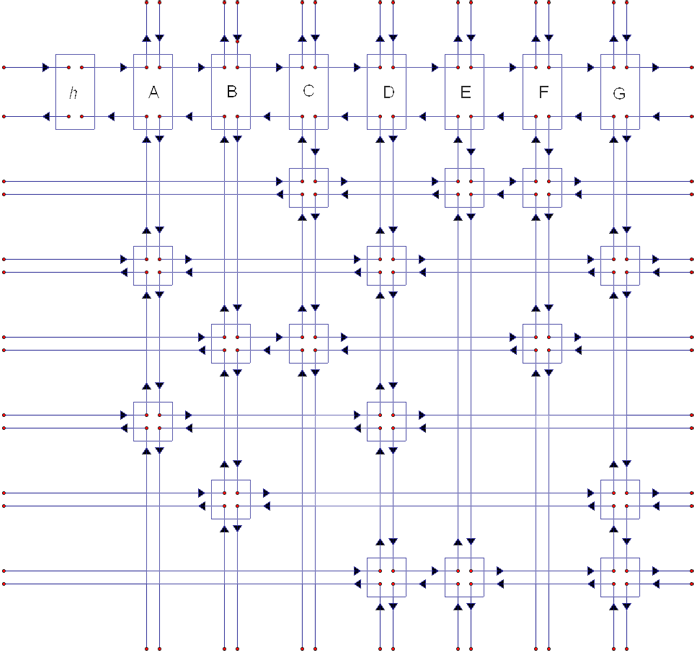The Algorithm

Given the ColumnNode h, the searching algorithm is then simplified to:

if( h.getRight() == h ) {
printSolution();
return;
}
else {
ColumnNode column = chooseNextColumn();
cover(column);

for( Node row = column.getDown() ; rowNode != column ; rowNode = rowNode.getDown() ) {

for( Node rightNode = row.getRight() ; otherNode != row ; rightNode = rightNode.getRight() )
cover( rightNode );

Search( k+1);

solutions.remove( rowNode );
column = rowNode.getColumn();

for( Node leftNode = rowNode.getLeft() ; leftNode != row ; leftNode = leftNode.getLeft() )
uncover( leftNode );
}

uncover( column );
}

Cover

cover( ColumnNode c ) This function is the crux of the algorithm. It removes a column from the matrix as well as remove all rows in the column from other columns they are in. The code becomes:

Node column = dataNode.getColumn();

column.getRight().setLeft( column.getLeft() );
column.getLeft().setRight( column.getRight() );

for( Node row = column.getDown() ; row != column ; row = row.getDown() )
for( Node rightNode = row.getRight() ; rightNode != row ; rightNode = rightNode.getRight() ) {
rightNode.getUp().setDown( rightNode.getDown() );
rightNode.getDown().setUp( rightNode.getUp() );
}
}

Note that we first remove the column from the other columns. Then we go down a column and remove the row by traversing the row to the right.

Let’s look at some illustrations. Here is the matrix before Column A is covered. All the links in bold are the links that are going to be affected by the cover function.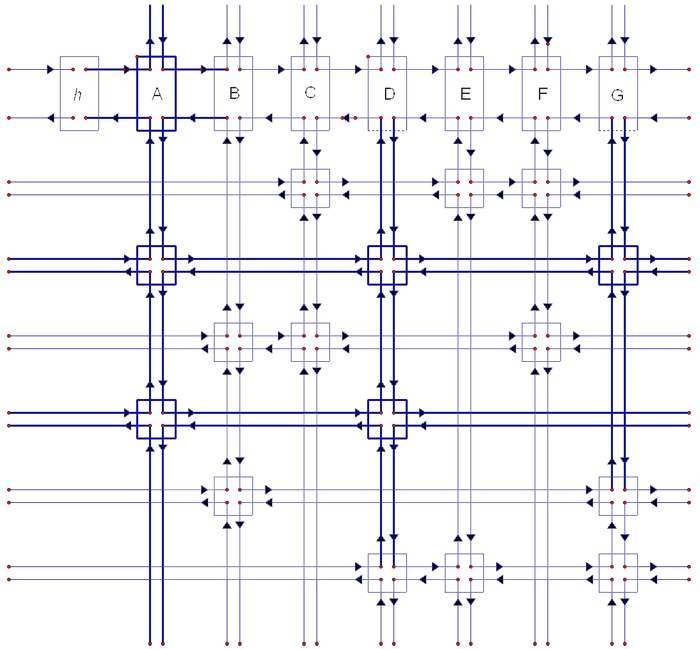Now here is the matrix after Column A has been covered. Notice how Column A and rows 2 and 4 are now independently linked outside of the matrix. In effect, they have been removed. Also note that each node that has been removed from the matrix still points to an element inside the matrix. This allows us to easily backtrack.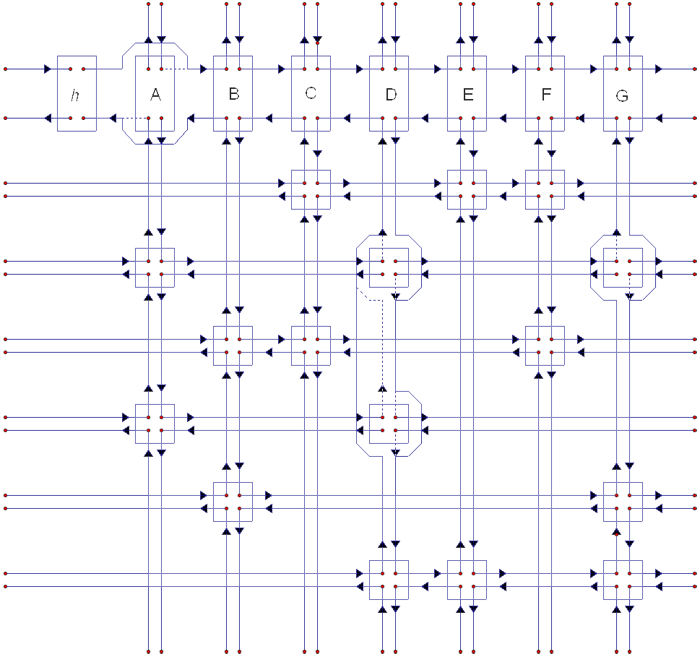Uncover

uncover( ColumnNode c ) This is the answer to easy backtracking. Taking advantage of the fact that every node that has been removed retains information about its neighbors, we can easily put the node back into the matrix using the reverse operation of cover.

Node column = dataNode.getColumn();

for( Node row = column.getUp() ; row != column ; row = row.getUp() )
for( Node leftNode = row.getleft() ; leftNode != row ; leftNode = leftNode.getRight() ) {
leftNode.getUp().setDown( leftNode.getDown() );
leftNode.getDown().setUp( leftNode.getUp() );
}
column.getRight().setLeft( column.getLeft() );
column.getLeft().setRight( column.getRight() );
}

Notice that the traversal through the column and row are opposite to that of cover. We first put the rows back by traveling up the column and to the left of the row. Then we put the column back. In effect, we undo the operation of cover.

Miscellaneous Functions

printSolution() takes all the rowNodes in the solution index, which is built by some data structure solutions and translates their positions in the matrix into the positions in the actual puzzle.

chooseNextColumn() advances the column pointer right or chooses the column with the least number of nodes. Choosing the column with the least number of nodes decreases the branching of the algorithm. It can be ignored if this isn’t needed.

Finding a Solution

A solution is found when all the columns have been removed from the matrix. This means that very row that we have added to the answer has one node in every column. All constraints have been satisfied by the set of rows.

A complete run-through of this algorithm is shown in file: Exact.Cover.Runthrough.xls.

Sudoku

So, what is Sudoku?

Sudoku is a logic puzzle. On a 9x9 grid with 3x3 regions, the digits 1-9 must be placed in each cell such that every row, column, and region contains only one instance of the digit. Placing the numbers is simply an exercise of logic and patience. Here is an example of a puzzle and its solution: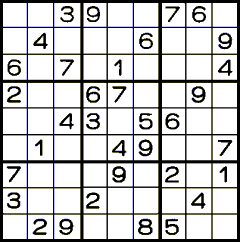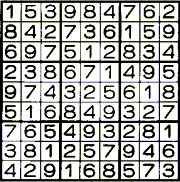Images from http://www.nikoli.co.jp/puzzles/1/index_text-e.htm

Applying the Exact Cover Problem to Sudoku

To create the sparse matrix of Sudoku needed to convert the problem into an Exact Cover Problem, we need to recognize what the rows and columns represent.

The columns represent the constraints of the puzzle. In Sudoku, we have four:

• A position constraint: Only 1 number can occupy a cell
• A row constraint: Only 1 instance of a number can be in the row
• A column constraint: Only 1 instance of a number can be in a column
• A region constraint: Only 1 instance of a number can be in a region

Each number comes with its own set of constraints. Therefore there are SIZE^2 * 4 columns., where SIZE is the number of candidates/rows/cols there are in the Sudoku Puzzle. In a 4x4, this would be 64 columns. In a 9x9, this would be 324 columns.

The rows represent every single possible position for every number. Therefore, there are SIZE ^ 3 rows. In a 4x4, this would be 64 columns. In a 9x9, this would be 729 rows. Each row would represent only one candidate position. Therefore, only 4 1s will be in the row, representing the constraints of that position.

The sparse matrix for a 4x4 Sudoku puzzle is seen in: 4x4.dlx.64x64.xls. The 9x9 sparse matrix is impractical to create by hand.

Given initial positions in the matrix, those rows will be included in the answer and covered. Then the Search algorithm will produce the solutions to the puzzle.

My Sudoku Solver

Having gone through 5 different revisions of the code, I have finally produced a program that can be presented. The following is a list of features of the program.

Features

Input
Currently, I have hardcoded Sudoku puzzles into 2D arrays. In the future, I may learn to made a GUI that allows the user to input numbers onto a Sudoku puzzle display.
Output
By default, the program will display the initial input puzzle and then the solution.
Graphics
The graphical element displays the doubly-linked lists in a linear fashion. Currently, it is only practical for 4x4 and 9x9 Sudoku puzzles.
Multiple Solutions
When this feature is enabled, the program can find multiple solutions to the puzzle given the input. It will continue to search until all possible attempts fail to find anymore solutions.
Alternate search method
As described in Knuth’s paper, there are two methods to choose the next column in the search method. The first simply chooses the node to the right of ColumnNode h. The second minimizes the branching of the algorithm by looking for the column with the least number of nodes in the column. The second method is much faster for solving 16x16 Sudoku puzzles. Both methods can be used in this program
Verbose and Debug Messages
When verbose messages are enabled, the program prints out the number of iterations search has been called and a snapshot of the current Sudoku puzzle. Debug messages allows the user to see what the program is doing.
Delay
A simply delay allows the graphical elements to catch up with the program, as well as slow down the output of the message. To see the real power of the program, set delay to 0 and do not enable messages and graphics.

Afterword

Dancing Links is a powerful algorithm that solves Exact Cover problems. These problems can lead to interesting algorithmic exercises such as the Pentominos problem, polyiamonds, tetrasticks, the N queens, traveling knight, and other chessboard derivatives. However, Exact Cover problems aren’t just theoretical mathematical puzzles though. They describe many real life problems: problems such as making hotel room assignments given a group that has a many specific room requests, and organizing simple flight schedules for airports. Dancing Links is a backtracking, depth-first algorithm that can find all possible solutions of the problem.

Credits

Dr. Donald Knuth and his lecture series: Computer Musings and his paper: Dancing Links
[http://www-cs-faculty.stanford.edu/~knuth/]
For his great work in Computer Algorithms and for discovering the art of Dancing Links.
Stanford University:       [http://www.stanford.edu/]
For educational possibilities through excellent professors
Stanford Center for Professional Development       [http://scpd.stanford.edu/scpd/default.htm]
For providing a link to Knuth’s Lecture Series at: [http://scpd.stanford.edu/scpd/students/Dam_ui/pages/ArchivedVideoList56K.asp?Include=musings]
Wikipedia       [http://en.wikipedia.org/wiki/Main_Page]
For providing basic information and useful links
Sudoku Programmer’s Forum       [http://www.setbb.com/phpbb/index.php]
For bringing together programmers to discuss various implementations of their programs
Stan Chesnutt       [http://www.bluechromis.com:8080/stan/chesnutt.html]
For his example of his Java implementation of a Sudoku Solver using the Dancing Links algorithm
Ruud van der Werf and his program SudoCue       [http://www.sudocue.net/]
For a simple visualization of the Dancing Links implementation and for his program to create random puzzles
Bob Hanson and his Sudoku Solver       [http://www.stolaf.edu/people/hansonr/Sudoku/]
For the visualization of the sparse matrix implementation of the Dancing Links algorithm
The American Computer Science League (ACSL)       [http://www.acsl.org/]
For providing the idea for this project
The Harker Research Symposium      [http://web.harker.org/WiSTEM/]
For providing me the opportunity to explore my passion for Computer Science
And finally my teacher, Dave Feinberg
[http://www.harker.org/page.cfm?p=160&dirid=29&dgid=1&dlid=1&keyword=dave]
For pushing me to enter the symposium